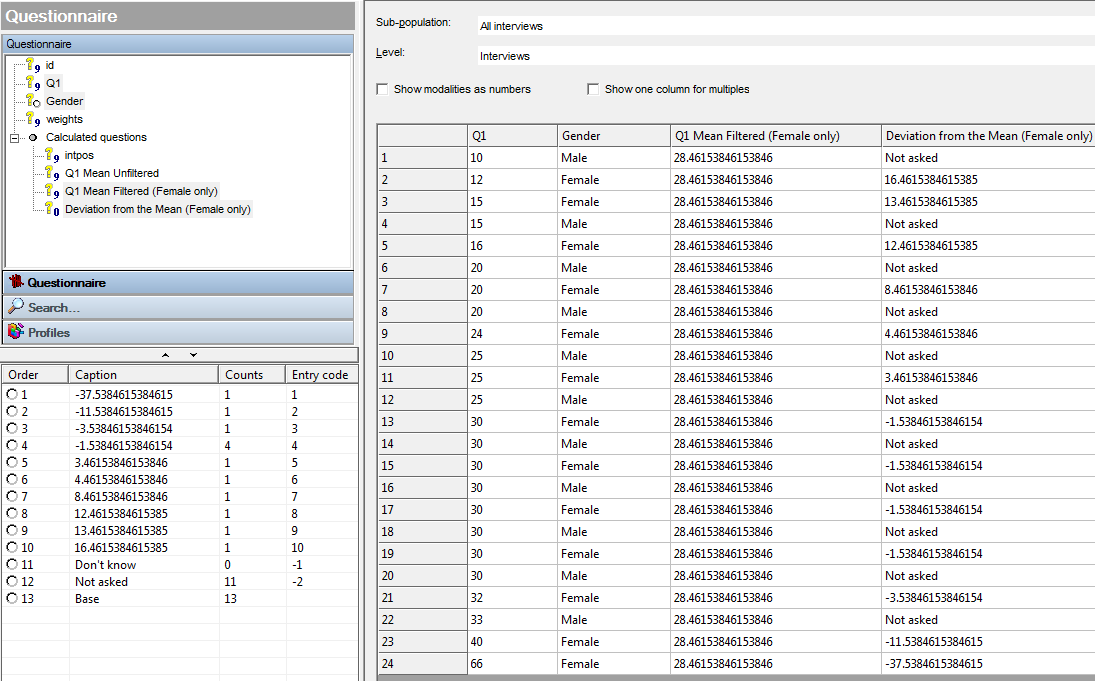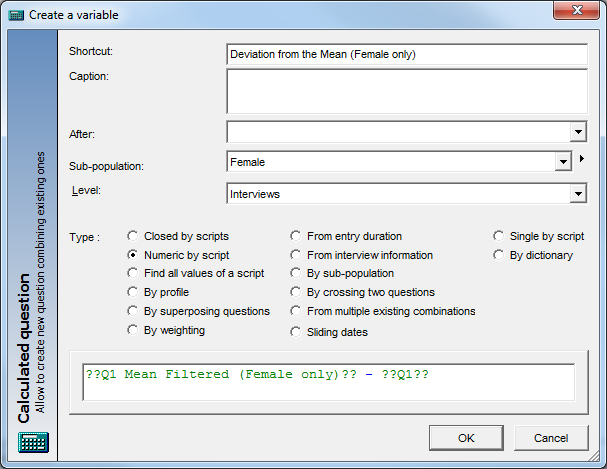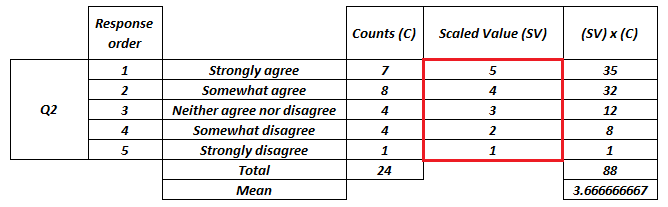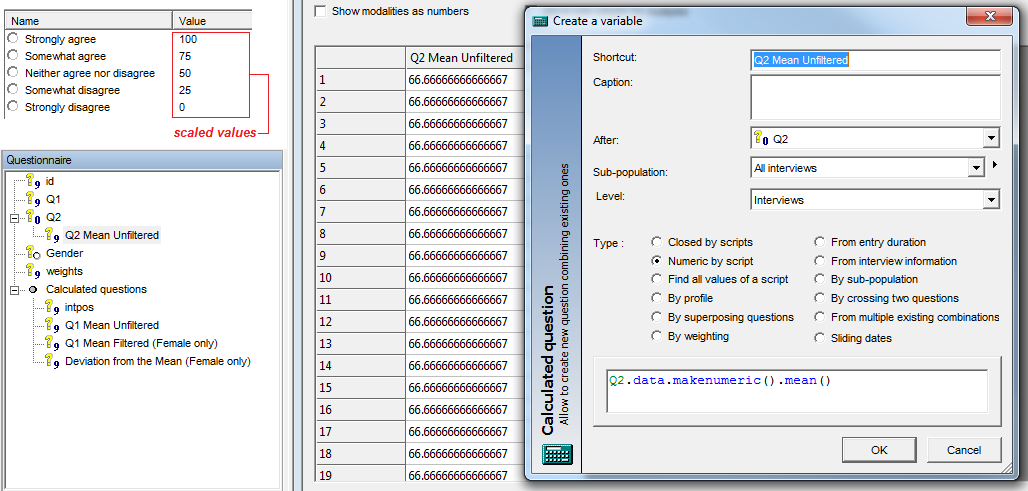Scroll

# Score Deviations from Mean Variable

Follow
 Summary This article is one in a series of articles that will go through examples of using aggregate script syntax new to Askia 5.3.5.0. Specifically, this article demonstrates how to calculate a filtered mean of a set of respondents, store it in a variable and then use it to create other variables for analysis. Applies to Askia Analyse. Written for Researchers, Data Processors, Analysts. Keywords Aggregated script, Mean, Filter, Sub-population, Deviation, Calculated variables, Numeric by script, Find all values of a script, Scaled responses, Scaled values

The .qes file and portfolio containing the example discussed is attached: Aggregated Scripts Examples 2.zip

Let’s imagine we are required  to find the mean rating for all females at Q1 and then create a numeric variable that stores their deviations from the mean i.e. how much above or below the mean their rating at Q1 is. We also require this to be updated automatically when new data is added.

We want to end up with a situation like this:We’ll start again by showing the simple syntax for an unfiltered mean:  Q1.data.mean()

Then we need syntax to filter out the men before we work out the mean:

```dim myVar = Q1.Data
dim myVar = myVar.Filter("Female")
myVar.mean()```

Now that we have our numeric variable which contains the mean and a numeric variable containing the ratings (Q1) we can subtract one from the other to find our deviations:In the syntax, the filter (sub-population) matches on name. In this version there is no option to define temporary / on-the-fly filters, however, this is under consideration for future versions of Analyse.Now let’s introduce another rating question, Q2. This question is a coded agreement scale.

If you try this: Q2.data.mean() you will see that no mean value is returned. Only ‘NR’. We have to apply another method to turn the coded variable into a numeric: Q2.data.makenumeric().mean().

You might still see NR returned as the value in your calculated variable when you use this syntax. If that’s the case then check that you have ‘Scaled responses’ selected for Q2 in Design. If you have selected ‘Scaled responses’ but not entered any scaled values then, by default, they are set as below.If you have set scaled values in Design then these will be used in the mean calculation. e.g. if I use 100, 75, 50, 25, 0 in Design then my mean using ‘Q2.data.makenumeric().mean()’ will return 66.66667.You have the ability to override the scaled value options in Design by changing the syntax to enter your own values. In Aggregated Scripts 2.qes I have no scaled values entered and don’t even have the ‘Scaled responses’ option ticked. However I can return a mean value in my calculated variable simply by using the syntax like this:

Q2.data.makenumeric(2,1,0,-1,-2,nr).mean()

This returns my mean of 0.6666666 without me having to go into Design and make any changes to the .qes file.